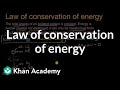# Class 11 Physics (India): Law of conservation of energy

Need more information? Get more details on the site of the provider.### Description

Sal discusses how energy can't be created or destroyed in an isolated system, and works an example of how energy is transformed when a ball falls toward the Earth.

## Class 11 Physics (India)

Let's learn, practice, and master topics of class 11 physics (NCERT) starting with kinematics and then moving to dynamics with Newton's laws of motion, work, energy, and power. Let's then use these as the foundation to learn about centre of mass, rotational motion, gravitation, solids, fluids, thermodynamics, and oscillations and waves.

## Conservation of energy

Learn about the law of conservation of energy, and how it can be useful for solving physics problems.

## Topic: Science

Learn about all the sciences, fr…

There are no frequently asked questions yet. If you have any more questions or need help, contact our customer service.

Didn't find what you were looking for? See also: Conservation, Physics, Law (General), C/C++, and English (FCE / CAE / CPE).

Sal discusses how energy can't be created or destroyed in an isolated system, and works an example of how energy is transformed when a ball falls toward the Earth.

## Class 11 Physics (India)

Let's learn, practice, and master topics of class 11 physics (NCERT) starting with kinematics and then moving to dynamics with Newton's laws of motion, work, energy, and power. Let's then use these as the foundation to learn about centre of mass, rotational motion, gravitation, solids, fluids, thermodynamics, and oscillations and waves.

## Conservation of energy

Learn about the law of conservation of energy, and how it can be useful for solving physics problems.

## Topic: Science

Learn about all the sciences, from physics, chemistry and biology, to cosmology and astronomy, across hundreds of videos, articles and practice questions.

There are no reviews yet.